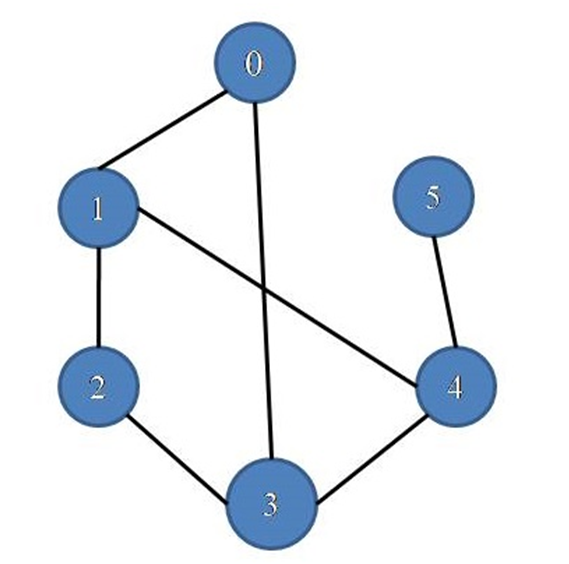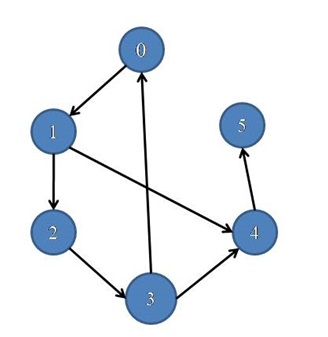• 有向循环图转换为环图What you will learn? 您将学到什么？ How to detect a cycle in an undirected graph? 如何检测向图中的循环？ In the graph below, 在下图中， It has cycles 0-1-4-3-0 or 0-1-2...
将有向循环图转换为无环图What you will learn?
您将学到什么？
How to detect a cycle in an undirected graph?
如何检测无向图中的循环？
In the graph below,
在下图中，It has cycles 0-1-4-3-0 or 0-1-2-3-0
它有个周期0-1-4-3-0或0-1-2-3-0
Algorithm:
算法：
Here we use a recursive method to detect a cycle in a graph.
在这里，我们使用递归方法来检测图中的循环 。
We check the presence of a cycle starting by each and every node at a time. 我们检查每个节点每次都开始一个循环的存在。
For each node
对于每个节点
Whenever we visited one vertex we mark it.
Except for the starting node, we store its parent node go for all its adjacent nodes.
If there is any marked vertex present except parent that means there is a cycle present in the graph.
Function:
功能：
Check(parent , current node){
visit[curr_node]=true;
for( all the adjacent vertices ){
if(not visited yet) then
check(current node, adjacent vertex);
else if( the vertex is not the parent) then
There is a cycle in the graph
}
}

C++ implementation:
C ++实现：
#include <bits/stdc++.h>
using namespace std;

void addedge(list<int>,int ,int );
void cycle_check(list<int>*,int);

//	Make a pair between vertex x and vertex y
void addedge(list<int> *ls,int x,int y){
ls[x].push_back(y);
ls[y].push_back(x);
return;
}

void check_cycle_util(list<int> *ls,bool *visit,int curr_node,int parent,int &temp){
visit[curr_node]=true;
list<int>::iterator it;
for(it=ls[curr_node].begin();it!=ls[curr_node].end();it++){
if(!visit[*it]){
check_cycle_util(ls,visit,*it,curr_node,temp);
}
else if(*it != parent && temp==0){
temp=1;
cout<<"There is a cycle in the graph\n";
break;
}
}
}

//checking the cycles in a graph
void cycle_check(list<int>*ls,int num){
bool *visit=new bool[num];
int temp=0;
for(int i=0;i<num;i++)
visit[i]=false;
for(int i=0;i<num;i++){
if(!visit[i] && temp==0){
check_cycle_util(ls,visit,i,-2,temp);
}
}
}

int main(){
int num;

cout<<"Enter the no. of vertices :";
cin>>num;

list<int> *ls=new list<int>[num];
addedge(ls,0,1);
addedge(ls,2,3);
addedge(ls,3,4);
addedge(ls,4,5);
addedge(ls,1,2);
addedge(ls,1,4);
addedge(ls,3,0);
cycle_check(ls,6);

return 0;
}

Output
输出量
Enter the no. of vertices : 6
There is a cycle in the graph


翻译自: https://www.includehelp.com/data-structure-tutorial/cycle-detection-in-an-undirected-graph.aspx将有向循环图转换为无环图
展开全文算法 java python leetcode c++
• 有向循环图转换为环图What to Learn? 学什么？ How to detect a cycle in a Directed graph? 如何在有向图中检测周期 ？ In the following graph, 在下图中， It has a cycle 0-1-2-3-0 它具有一个周期0-...
将有向循环图转换为无环图What to Learn?
学什么？
How to detect a cycle in a Directed graph?
如何在有向图中检测周期 ？
In the following graph,
在下图中，It has a cycle 0-1-2-3-0
它具有一个周期0-1-2-3-0
(1-2-3-4-1 is not cycle since edge direction is 1->4, not 4->1)
( 1-2-3-4-1不是循环的，因为边缘方向是1-> 4 ，而不是4-> 1 )
Algorithm:
算法：
Here we use a recursive method to detect a cycle in a graph.
在这里，我们使用递归方法来检测图中的循环 。
We check presence of a cycle starting by each and every node at a time. 我们检查每个节点一次开始的循环是否存在。
For each node
对于每个节点
Whenever we visited one vertex we mark it.
Except the starting node, we go for all its adjacent nodes.
If there is any marked vertex present that means there is a cycle present in the graph.
    Check(current node){
visit[curr_node]=true;
for( all the adjacent vertices ){
if(not visited yet) then
check(adjacent vertex);
else if( the vertex is already visited) then
There is a cycle in the graph
}
}

C++ implementation:
C ++实现：
#include <bits/stdc++.h>
using namespace std;

void addedge(list<int>,int ,int );
void cycle_check(list<int>*,int);

//	Make a pair between vertex x and vertex y
void addedge(list<int> *ls,int x,int y){
ls[x].push_back(y);
return;
}

void check_cycle_util(list<int> *ls,bool *visit,int curr_node,int &temp){
visit[curr_node]=true;
list<int>::iterator it;
for(it=ls[curr_node].begin();it!=ls[curr_node].end();it++){
if(!visit[*it]){
check_cycle_util(ls,visit,*it,temp);
}
else{
if(temp==0){
temp=1;
cout<<"There is a cycle in the graph\n";
break;
}
}
}
}
//checking the cycles in a graph
void cycle_check(list<int>*ls,int num){
bool *visit=new bool[num];
int temp=0;
for(int i=0;i<num;i++)
visit[i]=false;
for(int i=0;i<num;i++){
if(!visit[i] && temp==0){
check_cycle_util(ls,visit,i,temp);
}
}
}

int main(){
int num;
cout<<"Enter the no. of vertices :";
cin>>num;
list<int> *ls=new list<int>[num];
addedge(ls,0,1);
addedge(ls,2,3);
addedge(ls,3,4);
addedge(ls,4,5);
addedge(ls,1,2);
addedge(ls,1,4);
addedge(ls,3,0);
cycle_check(ls,6);

return 0;
}

Output
输出量
Enter the no. of vertices : 6
There is a cycle in the graph


翻译自: https://www.includehelp.com/data-structure-tutorial/cycle-detection-in-a-directed-graph.aspx将有向循环图转换为无环图
展开全文算法 java python leetcode c++
• 无向图的基类。图形用可选数据或属性存储节点和边。有无边。允许自循环，但不允许多个(平行)边。节点可以是具有可选键/值属性的任意(哈希)python对象。按照惯例 None 不用作节点。边缘表示为具有可选键/值属性的...
无向图的基类。图形用可选数据或属性存储节点和边。图有无向边。允许自循环，但不允许多个(平行)边。节点可以是具有可选键/值属性的任意(哈希)python对象。按照惯例 None 不用作节点。边缘表示为具有可选键/值属性的节点之间的链接。参数incoming_graph_data ( 输入图(可选，默认：无) )--要初始化图形的数据。如果没有(默认值)，则创建一个空图形。数据可以是to-networkx-graph()函数支持的任何格式，当前包括边缘列表、dict-of-dict、dict-of-list、networkx-graph、numpy-matrix或2d-ndarray、scipy-sparse-matrix或pygraphviz-graph。attr ( keyword arguments, optional (default= no attributes) )--作为键=值对添加到图中的属性。实际案例创建一个没有节点和边的空图形结构(“空图形”)。>>>G = nx.Graph()G可以通过几种方式生长。节点：一次添加一个节点：>>>G.add_node(1)添加任何容器中的节点(列表、dict、set，甚至是文件中的行或另一个图中的节点)。>>>G.add_nodes_from([2, 3])>>>G.add_nodes_from(range(100, 110))>>>H = nx.path_graph(10)>>>G.add_nodes_from(H)除了字符串和整数之外，任何可哈希的python对象(无对象除外)都可以表示一个节点，例如自定义的节点对象，甚至是另一个图。>>>G.add_node(H)边缘：G也可以通过添加边来增长。添加一个边缘，>>>G.add_edge(1, 2)边缘列表，>>>G.add_edges_from([(1, 2), (1, 3)])或者一组边缘，>>>G.add_edges_from(H.edges)如果某些边连接的节点还未在图形中，则会自动添加节点。添加已经存在的节点或边时没有错误。属性：每个图、节点和边都可以在关联的属性字典中保存键/值属性对(键必须是可哈希的)。默认情况下，这些值为空，但可以使用添加边、添加节点或直接操作分别命名为graph、node和edge的属性字典来添加或更改。>>>G = nx.Graph(day="Friday")>>>G.graph{'day': 'Friday'}使用add_node()、add_nodes_from()或g.nodes添加节点属性。>>>G.add_node(1, time="5pm")>>>G.add_nodes_from(, time="2pm")>>>G.nodes{'time': '5pm'}>>>G.nodes["room"] = 714  # node must exist already to use G.nodes>>>del G.nodes["room"]  # remove attribute>>>list(G.nodes(data=True))[(1, {'time': '5pm'}), (3, {'time': '2pm'})]使用add_edge()、add_edges_from()、下标符号或g.edges添加边缘属性。>>>G.add_edge(1, 2, weight=4.7)>>>G.add_edges_from([(3, 4), (4, 5)], color="red")>>>G.add_edges_from([(1, 2, {"color": "blue"}), (2, 3, {"weight": 8})])>>>G["weight"] = 4.7>>>G.edges[1, 2]["weight"] = 4警告：我们通过 G.edges 一种只读的类似dict的结构。但是，您可以在例如 G.edges[1, 2] . 因此，使用2组括号添加/更改数据属性： G.edges[1, 2]['weight'] = 4 (对于多图表： MG.edges[u, v, key][name] = value )捷径：许多常见的图形功能允许Python语法加速报告。>>>1 in G  # check if node in graphTrue>>>[n for n in G if n < 3]  # iterate through nodes[1, 2]>>>len(G)  # number of nodes in graph5通常，遍历图的所有边的最佳方法是通过邻居。据报道，邻居们都是邻接听写的。 G.adj 或 G.adjacency()>>>for n, nbrsdict in G.adjacency():...    for nbr, eattr in nbrsdict.items():...        if "weight" in eattr:...            # Do something useful with the edges...            pass但是，edges()方法通常更方便：>>>for u, v, weight in G.edges.data("weight"):...    if weight is not None:...        # Do something useful with the edges...        pass报告：使用对象属性和方法获得简单的图形信息。报告通常提供视图而不是容器，以减少内存使用。视图会随着图形的更新而更新，类似于dict视图。对象 nodes ， edges 和 adj 通过查找提供对数据属性的访问(例如 nodes[n] ， edges[u, v] ， adj[u][v] )和迭代(例如 nodes.items() ， nodes.data('color') ， nodes.data('color', default='blue') 同样地 edges )视图存在于 nodes ， edges ， neighbors() 'adj'和 degree .有关这些方法和其他杂项方法的详细信息，请参见下文。子类(高级)：graph类使用dict数据结构的dict of dict。外部dict(节点dict)保存由节点键控的相邻信息。下一个dict(adjlist-dict)表示相邻信息，并保存由neighbor键控的边缘数据。内部dict(边缘attr u dict)表示边缘数据，并保存由属性名称键控的边缘属性值。这三个dict中的每一个都可以在子类中被用户定义的dict-like对象替换。一般来说，应该维护类似dict的功能，但是可以添加额外的功能。要替换其中一个dict，请通过更改类(！)创建一个新的graph类。变量为该dict-like结构保留工厂。变量名为node洹dict_factory、node洹attr_dict_factory、adjlist_inner洹dict_factory、adjlist_outer洹dict_factory、edge洹attr_dict_factory和graph洹attr_dict_factory。node_dict_factory函数(默认值：dict)工厂函数，用于创建包含节点属性的dict，由节点ID键控。它不需要参数，并返回类似dict的对象节点u attr_dict_factory:函数，(默认值：dict)用于创建节点属性dict的factory函数，其中保存由属性名键入的属性值。它不需要参数，并返回类似dict的对象adjlist_outer_dict_factory函数(默认值：dict)factory函数用于在数据结构中创建最外部的dict，其中保存由节点键控的相邻信息。它不需要参数，并返回类似dict的对象。adjlist_inner_dict_factory函数(默认值：dict)factory函数用于创建邻接列表dict，其中保存由邻居键入的边缘数据。它不需要参数并返回类似dict的对象edge_attr_dict_factory函数(默认值：dict)用于创建边缘属性dict的工厂函数，它保存由属性名键入的属性值。它不需要参数，并返回类似dict的对象。graph_attr_dict_factory函数(默认值：dict)用于创建图形属性dict的factory函数，其中保存由属性名键入的属性值。它不需要参数，并返回类似dict的对象。通常，如果扩展不影响数据结构，则所有方法都将继承而不会出现问题，除非： to_directed/to_undirected . 默认情况下，这些方法创建一个有向图/图形类，您可能希望它们创建一个有向图/图形的扩展。为了方便这一点，我们定义了两个类变量，您可以在子类中设置这些变量。to_directed_class可调用(默认：有向图或多图)类以在 to_directed 方法。如果 None ，使用networkx类(有向图或多图)。to_undirected_class可调用(默认：图形或多图形)类以在 to_undirected 方法。如果 None ，使用networkx类(图形或多图形)。实际案例创建一个内存不足的图形类，通过对所有边使用单个属性dict来有效地禁用边属性。这会减少使用的内存，但会丢失边缘属性。>>>class ThinGraph(nx.Graph):...    all_edge_dict = {"weight": 1}......    def single_edge_dict(self):...        return self.all_edge_dict......    edge_attr_dict_factory = single_edge_dict>>>G = ThinGraph()>>>G.add_edge(2, 1)>>>G{'weight': 1}>>>G.add_edge(2, 2)>>>G is GTrue请看 ordered 有关通过重写基类创建图子类的更多示例 dict 像字典一样的东西。
展开全文• 有向无解决casbin循环继承的问题
背景
在casbin学习记录一文中,
我提到了casbin多重继承的功能.
这里多重继承是说, 比如对某个资源R1, 在: grouping policy: g2 = _, _的
设计下
如下的实际数据表示: r1同时属于r2和r3这两个分组
g2, r1, r2
g2, r1, r3,

问题提出
那么我们很自然就会有一个问题, 如果循环了怎么办?
比如:
g2, r1, r2
g2, r2, r3,
g2, r3, r1

上面的分组关系就是一个环, 很明显, 现实生活中是不可能有这种关系的, 而且这种数据也没有意义.
所以我们要避免这种情况.
问题分析
其实仔细想想, 这种多继承的关系, 其实就是一个有向图, 那问题就变成了, 如果保证有向图无环?
问题解决
这是个经典的有向图检测环的算法, 我是这样做的:

在存储新policy(g2, r1, r2就是一个policy)之前, 先取出已有的policy, 记录exist_policy,
其结构为: []string
将要存储的, 记为input_policy, 其结构为: []string
将exist_policy和input_policy认为是有向图的许多条边, 构建一个有向图.
对于input_policy的点进行检测(因为如果有环, input_policy的某些点肯定在环上).
判断有没有环

代码
下面是我写的有向图判断环的代码, 有一些方法和判断环无关的, 可不用管
package algo

import (
"bytes"
"container/list"
"fmt"
"strings"
)

// 有向图
type dGraph struct {
v int // 顶点数
e int // 边数

// 存储顶点之间关系的结构, 邻接表
m map[string][]string

// "谁指向它", 如edgeTo["2"] = "1", 表示1指向2
// 直观理解为: "2"的"前驱"节点是"1"
edgeTo map[string]string

// 是否在某次递归的栈里
onStask map[string]bool

// 从key出发的环
// 如: 1 => [1, 2, 3, 1]
circles map[string][]string

// 遍历过程中已经访问过的点标记下
visited map[string]bool
}

// m: 已经有的k,v表示的图, m为空表示创建一个空的图
func NewGraph() *dGraph {
return &dGraph{
m:       make(map[string][]string),
circles: make(map[string][]string),
visited: make(map[string]bool),
onStask: make(map[string]bool),
edgeTo:  make(map[string]string),
}
}

func (g *dGraph) Destroy() {
g.m = nil
g.edgeTo = nil
g.onStask = nil
g.circles = nil
g.visited = nil
}

// 数据不变, 记录的状态重置
func (g *dGraph) ResetStatus() {
g.circles = make(map[string][]string)
g.visited = make(map[string]bool)
g.onStask = make(map[string]bool)
g.edgeTo = make(map[string]string)
}

// 顶点数
func (g *dGraph) V() int {
slice := make([]string, 0)
for k, v := range g.m {
slice = append(slice, k)
slice = append(slice, v...)
}

marked := make(map[string]bool)
sum := 0
for _, v := range slice {
if !marked[v] {
marked[v] = true
sum++
}
}

return sum
}

// 边数
func (g *dGraph) E() int {
return g.e
}

// 添加一条边(v => w)
func (g *dGraph) AddEdge(v, w string) {
// 不能自成环
if v == w {
return
}

// 不接收空串
if v == "" || w == "" {
return
}

for _, u := range g.m[v] {
// w已经是v的邻接点了, 不要重复添加
if u == w {
return
}
}

if len(g.m[v]) == 0 { // v还没有邻接点
g.m[v] = make([]string, 0)
}
g.m[v] = append(g.m[v], w)

// 只要添加节点成功, 边数都是增加1
g.e++
}

// 返回顶点v的邻接点
func (g *dGraph) Adj(v string) []string {
return g.m[v]
}

// 以顶点v为起点进行深度优先遍历
// stopOnErr: 遍历过程中遇到错误(由visitor的返回值决定是否出错)立即停止遍历
func (g *dGraph) Dfs(v string, visitor func(v string) error, stopOnErr bool) {
var err error

err = visitor(v)
g.visited[v] = true
if err != nil && stopOnErr {
return
}

adjs := g.Adj(v)
for _, w := range adjs {
if !g.visited[w] {
g.Dfs(w, visitor, stopOnErr)
}
}
}

// 检测从v出发是否有环
func (g *dGraph) dfs4Circle(v string) {
g.onStask[v] = true // v在此次递归的栈里
g.visited[v] = true

// 如果从某顶点出发有多个环, 只记录一个
if len(g.circles[v]) > 0 {
return
}

adjs := g.Adj(v)
for _, w := range adjs {
if !g.visited[w] {
g.edgeTo[w] = v
g.dfs4Circle(w)
} else if g.onStask[w] {

stack := list.New()
// 如果图中无环的话, w(v的邻接点)在dfs过程中是不可能在onStask中的, 所以到这里说明成环
for x := v; x != w && len(x) > 0; x = g.edgeTo[x] {
stack.PushFront(x)
}
stack.PushFront(w)
stack.PushFront(v)

circle := make([]string, 0, stack.Len())
for e := stack.Front(); e != nil; e = e.Next() { // 出栈
circle = append(circle, e.Value.(string))
}

g.circles[w] = circle
return
}
}

// 某个点再无邻接点, 本次递归退出(针对v的深度优先结束)
// 重置 "在栈状态"
g.onStask[v] = false
}

// 从v出发的环
func (g *dGraph) Circle(v string) []string {
g.ResetStatus()
g.dfs4Circle(v)
return g.circles[v]
}

// 图中的所有环
func (g *dGraph) CirclesInGraph() map[string][]string {
g.ResetStatus()
visited := make(map[string]bool)

for k := range g.m {
if !visited[k] {
g.dfs4Circle(k)
visited[k] = true
}
}

// TODO: 环没有去重, 如: 1,2,3,1和2,3,1,2是一个环
return g.circles
}

// 按照邻接表的顺序打印图, 由于是map存储的起始顶点, 所以起始顶点的顺序不定
func (g *dGraph) String() string {
buffer := bytes.NewBufferString("")
for k, v := range g.m {
if _, err := buffer.WriteString(fmt.Sprintf("%s => [%s]\n", k, strings.Join(v, ","))); err != nil {
buffer.WriteString(fmt.Sprintf("write %s failed", v))
continue
}
}
return buffer.String()
}

测试代码
package algo

import (
"strings"
"testing"

"github.com/stretchr/testify/assert"
)

func TestGraph(t *testing.T) {
g := NewGraph()
if len(g.CirclesInGraph()) > 0 {
t.Fatalf("CirclesInGraph error")
}

g.AddEdge("1", "2")
g.AddEdge("2", "3")
g.AddEdge("3", "4")
g.AddEdge("4", "5")
g.AddEdge("4", "6")
g.AddEdge("8", "9")

// 测试重复数据
g.AddEdge("8", "9")

t.Logf(g:
%s, g.String())

path := make([]string, 0)
g.Dfs("1", func(v string) error {
path = append(path, v)
return nil
}, false)

g.ResetStatus()

expected := "1,2,3,4,5,6"
actual := strings.Join(path, ",")
if actual != expected {
t.Fatalf("expected: %v, actual: %v", expected, actual)
}

assert.Equal(t, 6, g.E(), "error edge num")
assert.Equal(t, 8, g.V(), "error vertex num")

// 检测环: 1,2,3,4,5,1
g.AddEdge("5", "1")
t.Logf(g:
%s, g.String())

t.Log(g.Circle("1"))
t.Log(g.CirclesInGraph())

// again
g.Destroy()
g = NewGraph()
g.AddEdge("1", "2")
g.AddEdge("2", "3")
g.AddEdge("2", "5")
g.AddEdge("3", "4")

g.AddEdge("4", "1")
if circle := g.Circle("4"); len(circle) == 0 {
t.Errorf("can not check circle for %v", "4")
}

if circle := g.Circle("1"); len(circle) == 0 {
t.Errorf("can not check circle for %v", "1")
}

// 两次输出结果可能不一样, 如1,2,3,1和2,3,1,2. 由于起点是map的key, 所以会有这种现象
t.Log(g.CirclesInGraph())
t.Log(g.CirclesInGraph())
}


欢迎补充指正!


展开全文• 有向无：为什么不能有环，有环会导致死循环。检查一个有向是否存在环要比无向复杂。(有向为什么比无向检查环复杂呢？)现实中管网会存在环吗？管网是有方向的，理论上也是无环的。arcgis有向无最短...
• osg场景图是一个有向无循环图，如下图所示： osg场景图中，所有节点全部为osg::Node或从其派生出来。 其中，叶子节点（osg::Geode）是模型数据节点（存放数据的节点），中间节点（或称枝节节点）为组节点（osg::...
• 给定一个已连接的无向图，请查找它是否包含任何循环。 例如，以下图形包含一个循环2–5–10–6–2： 推荐阅读： 1.使用BFS 当我们从v向图中的任何顶点进行广度优先搜索（BFS）时，我们可能会遇到一...
• 我希望能够找到有向图中的所有圈。在如果有向图中存在或不存在循环，以下代码将返回True或False：def cycle_exists(G):color = { u : "white" for u in G }found_cycle = [False]for u in G:if color[u] == ...
• 说明：对于dijkstra而言，是否有向判断方法几乎一致 思想：在迭代过程中，假设求出了i->j的最短路径，这是我们擦除这条边a，然后再次求i->j的最短路径b，此时如果b路径存在，那么a+b就是最小环的长度 Floyd求...1024程序员节
• 对于一个有向无，将至少存在一个入度为0的结点。显然，若不存在的话这将是一个有环。同时，最长路径的起点一定是入度为0的结点，我们就可以把所有入度为0的结点的far值设为0。入度不为0的far都设-1（方便起见...
• ## 判断无向图中是否有回路

万次阅读 多人点赞 2014-12-15 17:05:10
在有向图中，先找出入度为0的顶点，删除与这个顶点相关联的边（出边），将与这些边相关的其它顶点的入度减1，循环直到没有入度为0的定点。如果此时还有未被删除顶点，则必存在环路，否则不存在环路。C++ 遍历
• 以前只知道并查集可以判的环，Tarjan和dfs可以判有向图的环，有向图的最小环具体大小怎么求还真不太清楚； 目前可以知道的是Floyd可以求，但是复杂度为 O(n^3) ，不知道还有什么算法没有，这里先介绍Floyd...
• 所以这也是叫有向无的原因。 但是深度学习神经网络的计算不一样，是一种迭代式计算，像程序流程设计中的循环，会不断往复计算得到结果的误差率，误差率我们设置一个认为合适的值，当计算到在误差率内的时候，...大数据
• 一组实现向非循环图和一些相关功能的模块。 正在安装 要使用DAG模块，您需要做的就是安装@dags/core软件包： $yarn add @dags/core # or$ npm install @dags/core 用法 目前，该库提供以下模块： dag dag-...
• 一、定义： 在计算机科学领域，有向的拓扑排序是其顶点的线性排序，使得对于从顶点...如果且仅当图形没有定向循环，即如果它是有向无（DAG），则拓扑排序是可能的。 任何DAG具有至少一个拓扑排序，并且已知这...拓扑排序
• 的遍历 (graph traversal) 给出一个G和其中任意一个顶点V0， 从V0出发系统地访问G中所有的顶点， 每个顶点访问而且只访问一次 • 深度优先遍历 ... • 也可能会陷入死循环 , 如 存在回路的解决办法为每个顶...
• 现在一个没有边的简单无向图中只有一些孤立的顶点），顶点的标号从1开始。对图可以执行以下两种操作： 1 a b——用一条边连接两个顶点a和b。确保在执行此操作之前，两个顶点a和b之间没有直接连接的边。 2 k...
• 但是对于有向图，DFS不能直接求最大连通子图，因为两个节点之间并不是双向联通的，从a->b,不一定可以从b->a，这个时候我们介绍一种新的算法，tarjan tarjan() 首先我们考虑为什么一个会强连通，这是因为中...
• public void topoSort(){//仅仅针对有向图，基本思路是找到一个无后继的结点，将其删除，并放到排序数组的尾端，依次循环。直到没有结点。 int originalVertex = nVertex; while(nVertex > 0){ int ...拓扑排序 邻接矩阵
• ## 利用jquery写无缝循环滑动的轮播图

万次阅读 热门讨论 2016-04-22 23:14:11
1.效果gif： 2.主要实现思想： a.第一层div，设置overflow为hidden b.里面是一个ul，每个li里面个img或者为每个li设置背景图片也可以。 c.li设置为左浮动，排成一行，还有ul的宽度设置成li宽度的总和，...jquery 动画 轮播
• 这里记录一下无向图的广度优先遍历，无向图用邻接表表示，使用的的示例如下，关于的表示可以参照博客：无向图的表示：邻接矩阵和邻接表，这里不再赘述，无向图的表示的代码被封装到头文件queue.h 中。...c语言 遍历
• ## 无向图判断环

千次阅读 2013-04-15 09:08:17
方法：从一个顶点出发深度优先遍历可遍历所有结点，并且没有环或只有n-1条边。 若判断环：可以在遍历时...用拓扑排序不可，因为无向图各顶点都入度. 假设不存在自环与平行边 #include #include struct node
• 环的有向图可以排出一个拓扑顺序。首先将每个结点的入度求出（扫描一遍边表），如果没有入度为0的结点，则说明有环。在循环开始前，入度为0的结点表示其没有先修课程，可以看作是已排好拓扑序。循环中，每次选择一...
• 对于无向图的编写，传统方法两种： 1、邻接矩阵法（适合边数多的无向图）。 2、邻接表法（适合边数较少的无向图）。 在我转载的两篇无向图编写的博客中已经详细介绍过了。 但是使用邻接表法时总感觉编程...算法 结构 c++
• 如何正确 detect cycle?...如果在循环的任何时刻，我们试图访问一个状态为 “1” 的节点，都可以说明环。 如何正确识别中 connected components 的数量? 添加任意点，探索所有能到达的点，探索
• 1065: 无向图的连通分量计算 题目链接-1065: 无向图的连通分量计算 解题思路 并查集或dfs并查集或dfs并查集或dfs 并查集并查集并查集：将能相互连通的点的集合赋予一个共同的父亲(即合并)，然后统计父亲的个数就行......